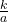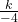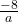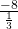## If t varies inversely as a and t= 2 when a = -4, what is t when a = 1/3? 1. -2 2/3 2. -24 3. – 1/6 4. -2/3

Question

If t varies inversely as a and t= 2 when a = -4, what is t when a = 1/3?

1. -2 2/3
2. -24
3. – 1/6
4. -2/3

in progress 0
5 months 2021-08-27T21:07:48+00:00 1 Answers 2 views 0

t = – 24

Step-by-step explanation:

Given t varies inversely as a then the equation relating them is

t =← k is the constant of variation

To find k use the condition t = 2 when a = – 4

2 =( multiply both sides by – 4 )

– 8 = k

t =← equation of variation

When a =, then

t == – 24